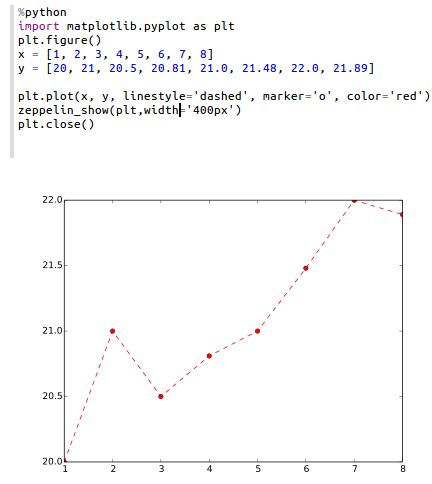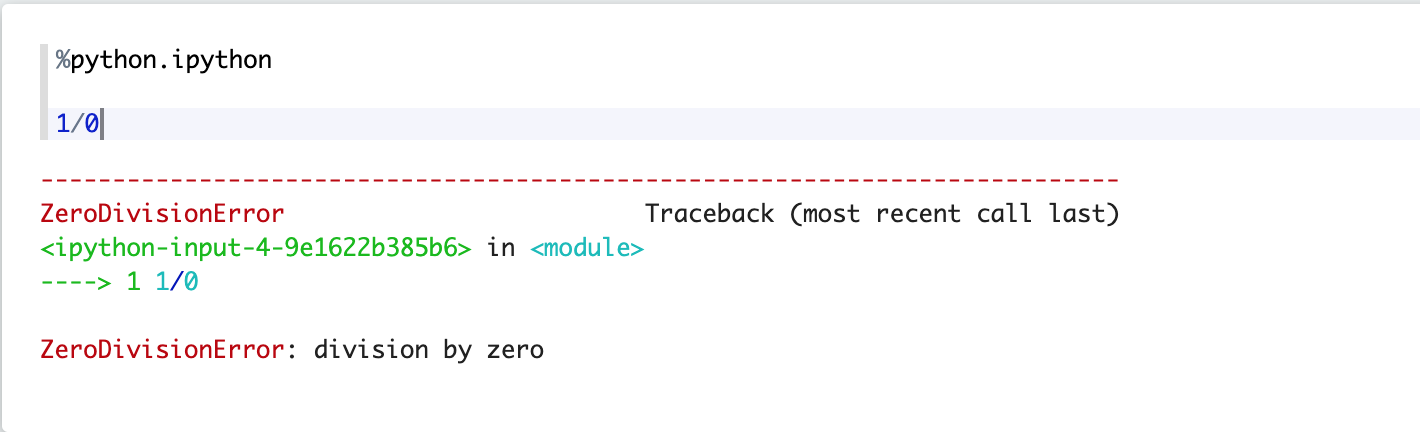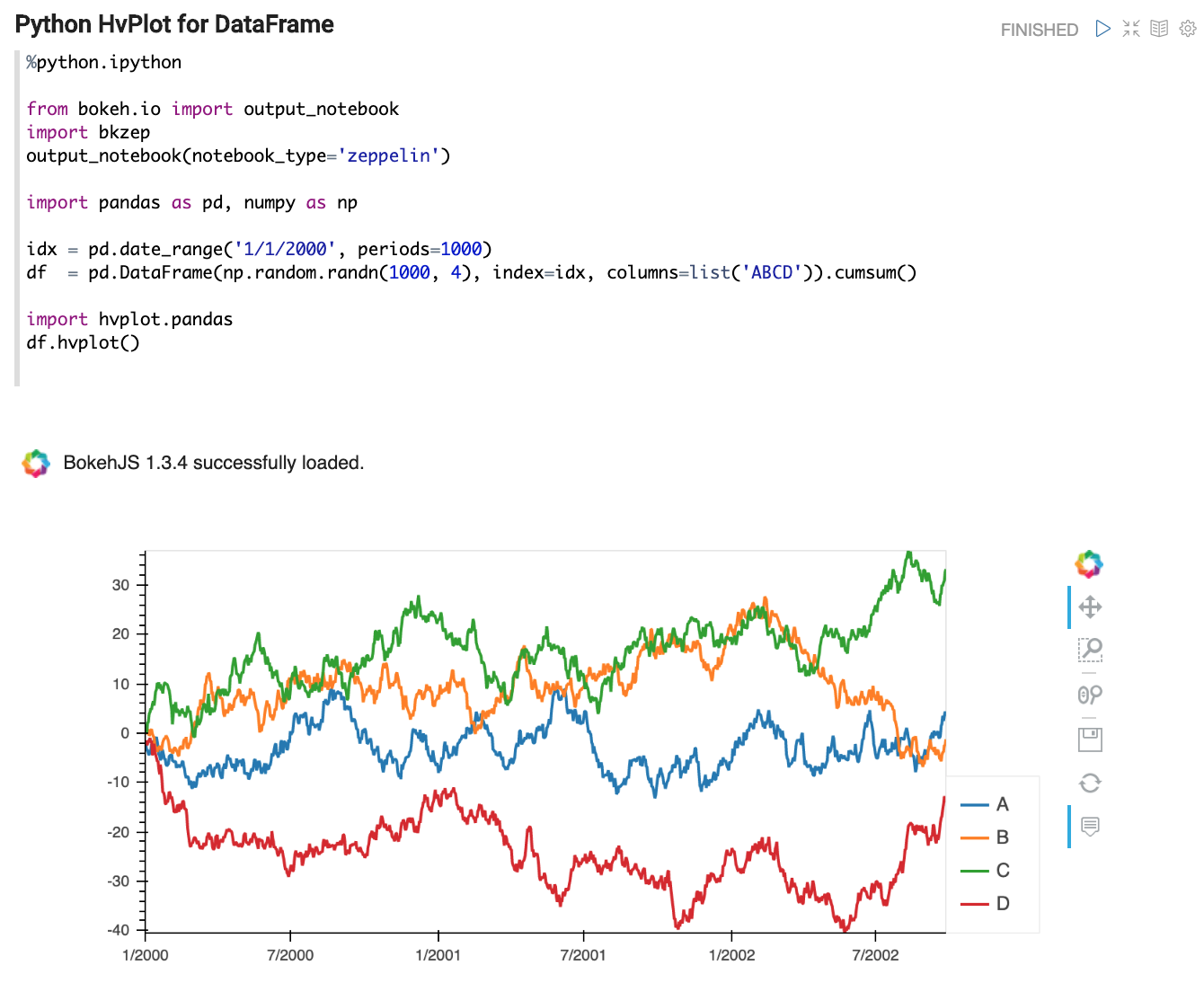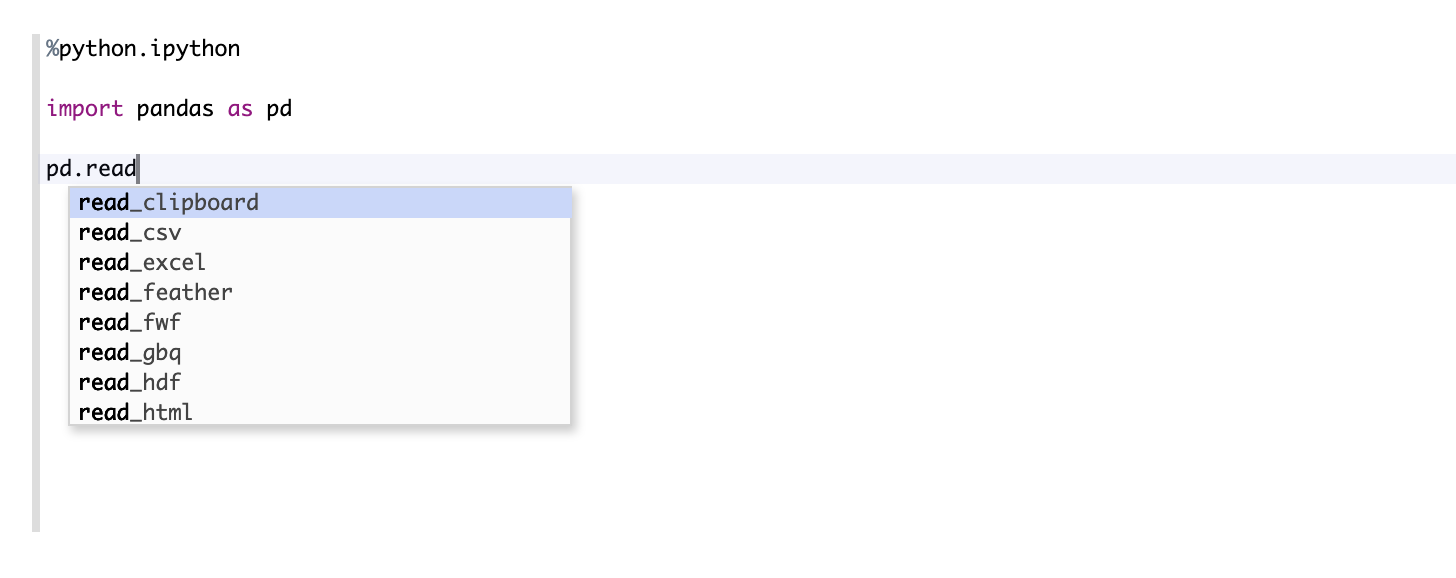# Python 2 & 3 Interpreter for Apache Zeppelin

## Overview

Zeppelin supports python language which is very popular in data analytics and machine learning.

Name Class Description
%python PythonInterpreter Vanilla python interpreter, with least dependencies, only python environment installed is required
%python.ipython IPythonInterpreter Provide more fancy python runtime via IPython, almost the same experience like Jupyter. It requires more things, but is the recommended interpreter for using python in Zeppelin, see below
%python.sql PythonInterpreterPandasSql Provide sql capability to query data in Pandas DataFrame via `pandasql`

## Configuration

Property Default Description
zeppelin.python python Path of the installed Python binary (could be python2 or python3). You should set this property explicitly if python is not in your `\$PATH`(example: /usr/bin/python).
zeppelin.python.maxResult 1000 Max number of dataframe rows to display.
zeppelin.python.useIPython true When this property is true, `%python` would be delegated to `%python.ipython` if IPython is available, otherwise IPython is only used in `%python.ipython`.

## Vanilla Python Interpreter (`%python`)

The vanilla python interpreter provides basic python interpreter feature, only python installed is required.

### Matplotlib integration

The vanilla python interpreter can display matplotlib figures inline automatically using the `matplotlib`:

``````%python

import matplotlib.pyplot as plt
plt.plot([1, 2, 3])
``````

The output of this command will by default be converted to HTML by implicitly making use of the `%html` magic. Additional configuration can be achieved using the builtin `z.configure_mpl()` method. For example,

``````z.configure_mpl(width=400, height=300, fmt='svg')
plt.plot([1, 2, 3])
``````

Will produce a 400x300 image in SVG format, which by default are normally 600x400 and PNG respectively. In the future, another option called `angular` can be used to make it possible to update a plot produced from one paragraph directly from another (the output will be `%angular` instead of `%html`). However, this feature is already available in the `pyspark` interpreter. More details can be found in the included "Zeppelin Tutorial: Python - matplotlib basic" tutorial notebook.

If Zeppelin cannot find the matplotlib backend files (which should usually be found in `\$ZEPPELIN_HOME/interpreter/lib/python`) in your `PYTHONPATH`, then the backend will automatically be set to agg, and the (otherwise deprecated) instructions below can be used for more limited inline plotting.

If you are unable to load the inline backend, use `z.show(plt)`:

``````%python

import matplotlib.pyplot as plt
plt.figure()
(.. ..)
z.show(plt)
plt.close()
``````

The `z.show()` function can take optional parameters to adapt graph dimensions (width and height) as well as output format (png or optionally svg).

``````%python

z.show(plt, width='50px')
z.show(plt, height='150px', fmt='svg')
``````## IPython Interpreter (`%python.ipython`) (recommended)

IPython is more powerful than the vanilla python interpreter with extra functionality. You can use IPython with Python2 or Python3 which depends on which python you set in `zeppelin.python`.

For non-anaconda environment

Prerequisites

``````- Jupyter `pip install jupyter`
- grpcio `pip install grpcio`
- protobuf `pip install protobuf`
``````

For anaconda environment (`zeppelin.python` points to the python under anaconda)

Prerequisites

``````- grpcio `pip install grpcio`
- protobuf `pip install protobuf`
``````

In addition to all the basic functions of the vanilla python interpreter, you can use all the IPython advanced features as you use it in Jupyter Notebook.

e.g.

### Use IPython magic

``````%python.ipython

#python help
range?

#timeit
%timeit range(100)
``````

### Use matplotlib

``````%python.ipython

%matplotlib inline
import matplotlib.pyplot as plt

print("hello world")
data=[1,2,3,4]
plt.figure()
plt.plot(data)
``````

### Colored text output### More types of visualization

e.g. IPython supports hvplot### Better code completionBy default, Zeppelin would use IPython in `%python` if IPython prerequisites are meet, otherwise it would use vanilla Python interpreter in `%python`. If you don't want to use IPython via `%python`, then you can set `zeppelin.python.useIPython` as `false` in interpreter setting.

## Pandas integration

Apache Zeppelin Table Display System provides built-in data visualization capabilities. Python interpreter leverages it to visualize Pandas DataFrames though similar `z.show()` API, same as with Matplotlib integration.

Example:

``````%python

import pandas as pd
z.show(rates)
``````

## SQL over Pandas DataFrames

There is a convenience `%python.sql` interpreter that matches Apache Spark experience in Zeppelin and enables usage of SQL language to query Pandas DataFrames and visualization of results though built-in Table Display System.

Prerequisites

• Pandas `pip install pandas`
• PandaSQL `pip install -U pandasql`

Here's one example:

• first paragraph

``````%python
``````

import pandas as pd rates = pd.read_csv("bank.csv", sep=";") ```

• next paragraph

``````%python.sql
``````

SELECT * FROM rates WHERE age < 40 ```

## Using Zeppelin Dynamic Forms

You can leverage Zeppelin Dynamic Form inside your Python code.

Example :

``````%python

### Input form
print(z.input("f1","defaultValue"))

### Select form
print(z.select("f2",[("o1","1"),("o2","2")],"o1"))

### Checkbox form
print("".join(z.checkbox("f3", [("o1","1"), ("o2","2")],["o1"])))
``````

## ZeppelinContext API

Python interpreter create a variable `z` which represent `ZeppelinContext` for you. User can use it to do more fancy and complex things in Zeppelin.

API Description
z.put(key, value) Put object `value` with identifier `key` to distributed resource pool of Zeppelin, so that it can be used by other interpreters
z.get(key) Get object with identifier `key` from distributed resource pool of Zeppelin
z.remove(key) Remove object with identifier `key` from distributed resource pool of Zeppelin
z.getAsDataFrame(key) Get object with identifier `key` from distributed resource pool of Zeppelin and converted into pandas dataframe. The object in the distributed resource pool must be table type, e.g. jdbc interpreter result.
z.angular(name, noteId = None, paragraphId = None) Get the angular object with identifier `name`
z.angularBind(name, value, noteId = None, paragraphId = None) Bind value to angular object with identifier `name`
z.angularUnbind(name, noteId = None) Unbind value from angular object with identifier `name`
z.show(p) Show python object `p` in Zeppelin, if it is pandas dataframe, it would be displayed in Zeppelin's table format, others will be converted to string
z.textbox(name, defaultValue="") Create dynamic form Textbox `name` with defaultValue
z.select(name, options, defaultValue="") Create dynamic form Select `name` with options and defaultValue. options should be a list of Tuple(first element is key, the second element is the displayed value) e.g. `z.select("f2",[("o1","1"),("o2","2")],"o1")`
z.checkbox(name, options, defaultChecked=[]) Create dynamic form Checkbox `name` with options and defaultChecked. options should be a list of Tuple(first element is key, the second element is the displayed value) e.g. `z.checkbox("f3", [("o1","1"), ("o2","2")],["o1"])`
z.noteTextbox(name, defaultValue="") Create note level dynamic form Textbox
z.noteSelect(name, options, defaultValue="") Create note level dynamic form Select
z.noteCheckbox(name, options, defaultChecked=[]) Create note level dynamic form Checkbox
z.run(paragraphId) Run paragraph
z.run(noteId, paragraphId) Run paragraph
z.runNote(noteId) Run the whole note

## Python environments

### Default

By default, PythonInterpreter will use python command defined in `zeppelin.python` property to run python process. The interpreter can use all modules already installed (with pip, easy_install...)

### Conda

Conda is an package management system and environment management system for python. `%python.conda` interpreter lets you change between environments.

#### Usage

• get the Conda Information:

``````%python.conda info
``````
• list the Conda environments:

``````%python.conda env list
``````
• create a conda enviornment:

``````%python.conda create --name [ENV NAME]
``````
• activate an environment (python interpreter will be restarted):

``````%python.conda activate [ENV NAME]
``````
• deactivate

``````%python.conda deactivate
``````
• get installed package list inside the current environment

``````%python.conda list
``````
• install package

``````%python.conda install [PACKAGE NAME]
``````
• uninstall package

``````%python.conda uninstall [PACKAGE NAME]
``````

### Docker

`%python.docker` interpreter allows PythonInterpreter creates python process in a specified docker container.

#### Usage

• activate an environment

``````%python.docker activate [Repository]
%python.docker activate [Repository:Tag]
%python.docker activate [Image Id]
``````
• deactivate

``````%python.docker deactivate
``````

Here is an example

``````# activate latest tensorflow image as a python environment
%python.docker activate gcr.io/tensorflow/tensorflow:latest
``````

## Technical description

• Interrupt a paragraph execution (`cancel()` method) is currently only supported in Linux and MacOs. If interpreter runs in another operating system (for instance MS Windows) , interrupt a paragraph will close the whole interpreter. A JIRA ticket (ZEPPELIN-893) is opened to implement this feature in a next release of the interpreter.
• Progression bar in webUI (`getProgress()` method) is currently not implemented.